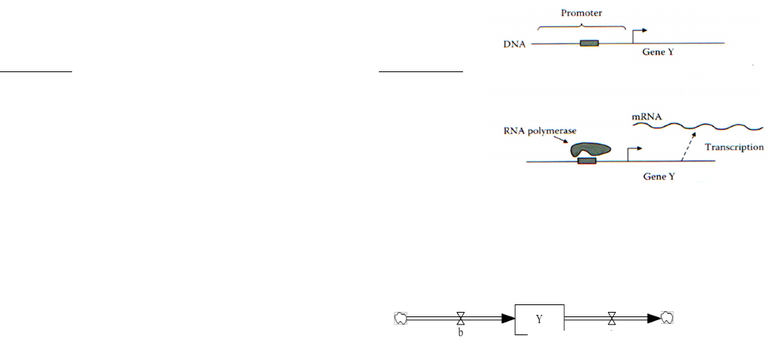# Biology 2382B Lecture Notes - Lac Operon, Stb, Repressor

16 views4 pagesGenetics Lecture No. 16: Quantitating Gene Regulation
Monday March 11th, 2013
Introduction:
-So far, we have examined gene regulation in a strictly qualitative manner (e.g. the
transcriptional levels of the lac operon were either high or low; the presence of glucose in the
growth media decreased transcription of the lac operon). Is it possible to examine the
transcription of genes in a quantitative manner? If so what could this tell us about transcriptional
regulatory networks?
Quantitating Gene Regulation:
-The goal of quantitating gene regulation is to create working
mathematical models of two very simple genetic networks. Here
is a model we can use for a given gene Y:
Activation: Repression:
X -> (+) Y
X -> (-) Y
X = activator X =
repressor Y = target gene
Y = target gene
-Using this model, we can quantify the rate of mRNA production and degradation for gene Y
using a “source and sink” model:
The Y mRNA is being accumulated in
box Y in nmoles:
1) When production rate >
degradation rate, accumulation of Y mRNA in the box. 2) When production
rate < degradation rate, loss of Y mRNA in the box. 3) When
production rate = degradation rate, levels of Y mRNA stay the same.
The level of Y mRNA is dependent on two things:
1) How much Y mRNA is produced through transcription of the source (rate of production)
(rate of degradation) Spigots (a and b) control the flow of Y mRNA into the box:
1) When spigot b is open, there is a high production rate of
Y mRNA (in nmoles/min) 2) When spigot a is open, there is a high
degradation rate of Y mRNA (1/min) Degradation rate depends on the amount of Y
mRNA that is present: If a = 0.1/min, 10% of Y mRNA is
degraded every minute If at an instant in
time, there are 1000 nmoles, the rate of degradation is 100 nmol/min If at
Sink
Source
dY/dt = b - aY
Unlock document

This preview shows page 1 of the document.
Unlock all 4 pages and 3 million more documents.